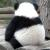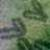## 关于在drawRect中的变量问题

Mrg 发布于 2013/02/27 14:30

a.h中有变量
{
int  x,y;
}
-(void)setXY:(int)xx Y:(int)yy;

a.m中的drawRect:(NSRect) rect
-(void)drawRect:(NSRect) rect
{
..........
x
y
....

}

-(void)setXY:(int)xx Y:(int)yy
{
x= xx;
y = yy;
}

0-(void)setXY:(int)xx Y:(int)yy

x= xx;
y = yy;
}

BTW:

0#### 引用来自“henius”的答案

-(void)setXY:(int)xx Y:(int)yy

x= xx;
y = yy;
}

BTW:

0#### 引用来自“henius”的答案

-(void)setXY:(int)xx Y:(int)yy

x= xx;
y = yy;
}

BTW:

int  x,y;

0#### 引用来自“henius”的答案

-(void)setXY:(int)xx Y:(int)yy

x= xx;
y = yy;
}

BTW:

int  x,y;

http://www.oschina.net/question/863564_91227